NEET  >  Bernoulli's Equation

# Bernoulli's Equation | Physics Class 11 - NEET

 1 Crore+ students have signed up on EduRev. Have you?

6. Bernoullis Equation

It relates the variables describing the steady laminer of liquid. It is based on energy conservation.

Assumptions

The fluid is incompressible, non-viscous, non rotational and streamline flow.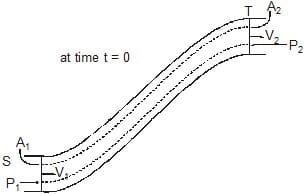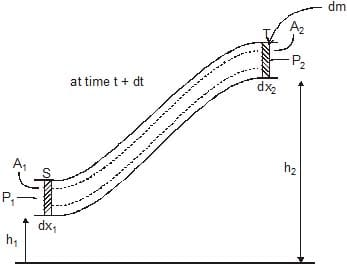Mass of the fluid entering from side S
dm1 = p A1 dX1 = p dV1
The work done in this displacement dx1 at point S is
Wp1 = F1dx1 = P1A1dx1
Wp1 = P1dV1    {∵ A1dx1 = dV1}
At the same time the amount of fluid moves out of the tube at point T is dm2 = pdV2

According to equation of coutniuity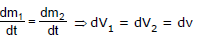The work done in the displacement of dm2 mass at point T

Wp2 - P2dV2

Now applying work energy theorem.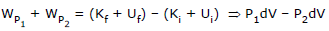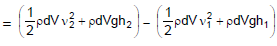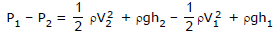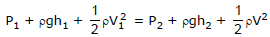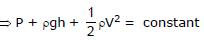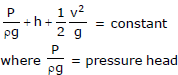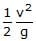= volume head

6.1 Application of Bernoullis principle

Magnus effect : When a spinning ball is thrown, it deviates from its usual path in flight. This effect is called Magnus effect and plays an important role in tennis, cricket and soccer, etc., as by applying appropriate spin the moving ball can be made to curve in any desired direction.

If a ball is moving from left to right and also spinning about a horizontal axis perpendicular to the direction of motion as shown in figure, then relative to the ball air will be moving from right to left.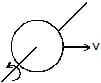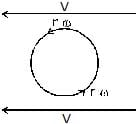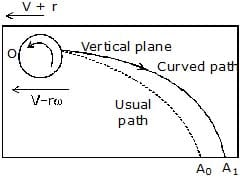(A)                                              (B)                                                   (C)

The resultant velocity of air above the ball will be (V rw) while below it (V -rw) (shown figure). So in accordance with Bernoulli's principle pressure above the ball will be less than below it. Due to this difference of pressure an upward force will act on the ball and hence the ball will deviate from its usual path OA0 and will hit the ground at A1 following the path OA1 (figure shown) i.e., if a ball is thrown with back spin, the pitch will curve less sharply prolonging the flight.

Similarly if the spin is clockwise, i.e., the ball is thrown with top-spin, the force due to pressure difference will act in the direction of gravity and so the pitch will curve more sharply shortening the flight.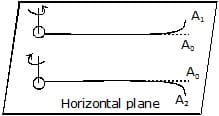Furthermore, if the ball is spinning about a vertical axis, the curving will be sideays as shown in figure. producing the so called out swing or in swing.

Action of Atomiser : The action of aspirator, carburettor, paint-gun, scent-spray or insect-sprayer is based on Bernoulli's principle. In all these by means of motion of a piston P in a cylinder C high speed air is passed over a tube T dipped in liquid L to be sprayed. High speed air creates low pressure over the tube due to which liquid (paint, scent, insecticide or petrol) rises in it and is then blown off in very small droplets with expelled air.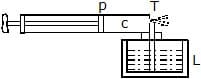Working of Aeroplane : This is also based on Bernouilli's principle. The wings of the aeroplane are having tapering as shown in figure. Due to this specific shape of wings when the aeroplane runs, air passes at higher speed over it as compared to its lower surface. This difference of air speeds above and below the wings, in accordance with Bernoulli's principle, creates a pressure difference, due to which an upward force called ' dynamic lift' ( = pressure difference × area of wing) acts on the plane. If this force becomes greater than the weight of the plane, the plane will rise up.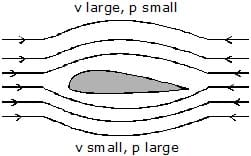Ex.15 If pressure and velocity at point A is P1 and V1 respectively & at point B is P2, V2 is the figure as shown. Comment on P1 and P2

Sol. From equation of continuity A1V1 = A2V2

here A1 > A2

⇒ V1 < V2 ....(1)

from Bernoulli's equation. We can write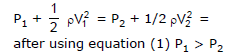Torricielli's Law of Efflux (Velocity of efflux)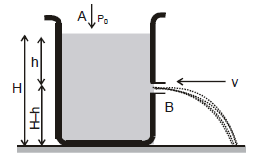Cross-sectional Area of hole at A is greater than B If water is come in tank with velocity vA and going out side with velocity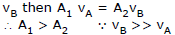on applying Bernoulli theorem at A and B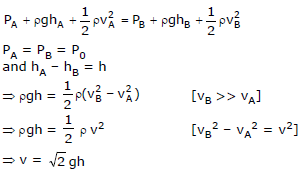Range (R)

Let us find the range R on the ground.

Considering the vertical motion of the liquid.

(H -h) =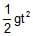or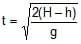Now, considering the horizontal motion,

R = vt or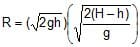or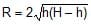From the expression of R, following conclusions can be drawn,

(i) Rh = RH -h

as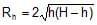and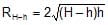This can be shown as in Figure

(ii) R is maximum at H/2 and Rmax = H.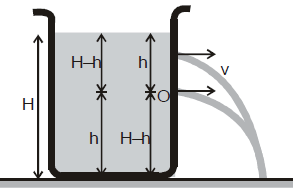Proof : R= 4 (Hh – h2)
For R to be maximum.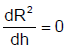or H - 2h = 0 or h = H/2

That is, R is maximum at h=H/2

and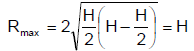Proved

Ex.16 A cylindrical dark 1 m in radius rests on a platform 5 m high. Initially the tank is filled with water up to a height of 5 m. A plug whose area is 10–4 m2 is removed from an orifice on the side of the tank at the bottom Calculate

(a) initial speed with which the water flows from the orifice

(b) initial speed with which the water strikes the ground and

(c) time taken to empty the tank to half its original volume

(d) Does the time to be emptied the tank depend upon the height of stand.

Sol. The situation is shown in figure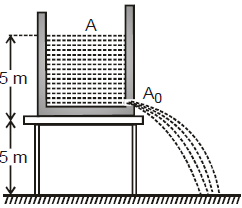(a) As speed of flow is given by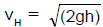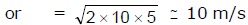(b) As initial vertical velocity of water is zero, so its vertical velocity when it hits the ground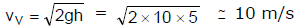So the initial speed with which water strikes the ground.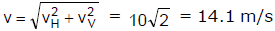(c) When the height of water level above the hole is y, velocity of flow will be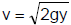and so rate of flow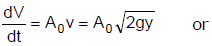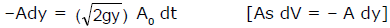Which on integration improper limits gives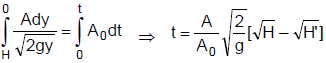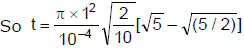= 9.2 × 103s – ~ 2.5 h

(d) No, as expression of t is independent of height of stand.

6.1 Venturimeter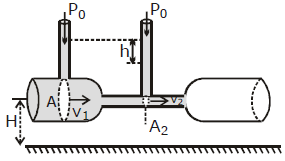Figure shows a venturimeter used to measure flow speed in a pipe of non - uniform cross-section. We apply Bernoulli's equation to the wide (point 1) and narrow (point 2) parts of the pipe, with h1 = h2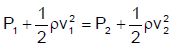From the continuity equation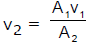Substituting and rearranging, we get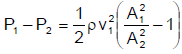Because A1 is greater than A2 , v2 is greater than v1 and hence the pressure P2 is less than P1. A net force to the right acceleration the fluid as it enters the narrow part of the tube (called throat) and a net force to the left slows as it leaves. The pressure difference is also equal to ρgh, where h is the difference in liquid level in the two tubes. Substituting in Eq. (1), we get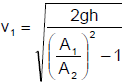6.2 Pivot Tube

It is a device used to measure flow velocity of fluid. It is a U shaped tube which can be inserted in a tube or in the fluid flowing space as shown in figure shown. In the U tube a liquid which is immiscible with the fluid is filled upto a level C and the short opening M is placed in the fluid flowing space against the flow so that few of the fluid particles entered into the tube and exert a pressure on the liquid in limb A of U tube. Due to this the liquid level changes as shown in the figure show.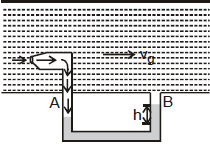At end B fluid is freely flowing, which exert approximately negligible pressure on this liquid. The pressure difference at ends A and B can be given by measuring the liquid level difference h as

It is a gas, then

PA - PB = ρhg

It if the liquid of density , then

PA - PB = h(ρ - ρg)g

Now if we apply Bernoulli's equation at the ends A and B we'll have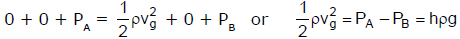Now by using equations, we can evaluate the velocity v, with which the fluid is flowing.

Note : Pivot tube is also used to measure velocity of aeroplanes with respect to wind. It can be mounted at the top surface of the plain and hence the velocity of wind can be measured with respect to the plane.

6.3 SIPHON

It is a pipe used to drain liquid at a lower height but the pipe initially rises and then comes down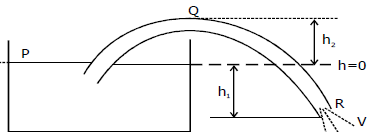let velocity of outflow is v and the pipe is of uniform cross-section A. Applying Bernoulli's Equation between P (top of tank) and R (Opening of pipe) we get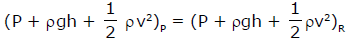⇒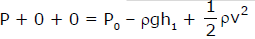here velocity is considered zero at P since area of tank is very large compared to the area of pipe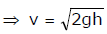Naturally for siphon to work

h> 0

Now as area of pipe is constant so by equation of continuity

as Av = constant so velocity of flow inside siphon is also constant between Q and R

(P + pgh + 1/2 pv2)Q = (P + pgh + 1/2 pv2)R ⇒ PQ + pgh2 = P0 - pgh1 (v is same)

⇒ PQ = P0 - Pg (h1 + h2) as PQ = 0    ⇒ P0 > pg (h1 + h2) means (h1 + h2) should not be more than P/pg for siphon to work

The document Bernoulli's Equation | Physics Class 11 - NEET is a part of the NEET Course Physics Class 11.
All you need of NEET at this link: NEET

## Physics Class 11

124 videos|464 docs|210 tests

## Physics Class 11

124 videos|464 docs|210 tests

### How to Prepare for NEET

Read our guide to prepare for NEET which is created by Toppers & the best Teachers

Track your progress, build streaks, highlight & save important lessons and more!(Scan QR code)

,

,

,

,

,

,

,

,

,

,

,

,

,

,

,

,

,

,

,

,

,

;